/

### Enterprise Risk Management Formula Book 12. Copulas

12.1        Definition

A copula is a multivariate cumulative distribution function for andimensional random vector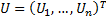in the unit hypercube (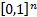) that has uniform marginals,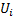, each distributed according to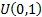but not in general independent of each other. Let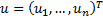also be restricted to the unit hypercube. Then a copula is defined as a function of the form: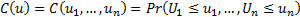Equivalently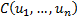is the joint cumulative distribution function for the random vector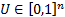.

The copula density (for a continuous copula) is the pdf for which the cdf is the copula.

12.2        Properties

In the bivariate case (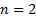) for a general function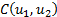to be a copula it must satisfy the following properties:

1.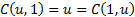for all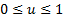2.must be increasing in both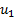and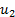3.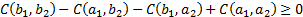for all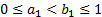and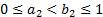4.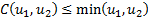5.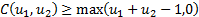12.3        Sklar’s theorem

If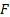is a joint (cumulative) distribution with marginal cdf’s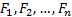then there exists a copula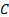which maps the unit hypercubeonto the interval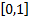such that for all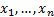we have: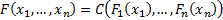Moreover, if the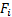are continuous functions then the copula is unique and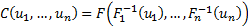Conversely, supposeis a copula and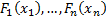are univariate cdf’s. Then the functionis a joint distribution function with marginal cdf’s.

12.4        Example copulas

The Archimedean family involves copulas of the following form, where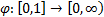,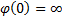,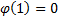,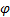is continuous and strictly decreasing and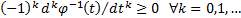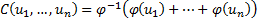Special cases include the Clayton copula which has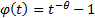(for some suitable value of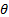) and the independence or product copula which has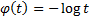.

12.5        Tail dependence

If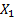and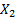are continuous random variables with copulathen their coefficient of (joint lower) tail dependence (if it exists) is: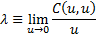For continuous random variables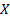and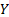each with lower limit of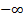the coefficient of (lower) tail dependence is also: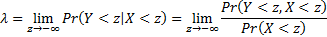12.6        Simulating copulas

Correlated Gaussian (i.e. multivariate normal) random variables (i.e. random variables with a Gaussian copula and Gaussian marginals) can be generated using Cholesky decomposition.

For random variables that have a Gaussian copula but non-normal marginal (with cdfs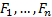) we can generate a vector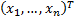of correlated Gaussian random variables as above and then transform as per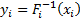.

In general, for non-Gaussian copulas we may need to generate a vector of unit uniform random variables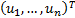and then transform them using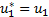,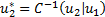etc.